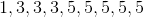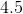# Algebra 1 : How to find mode

## Example Questions

### Example Question #111 : How To Find Mode

Find the mode of the following set of numbers:

1,1,3,7,7,8,9,12,15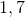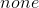Explanation:

To find the mode, you must find the most frequent number. Make sure that all of you numbers are grouped together so you can easily count them. In our given set, 3,8,9,12, and 15 all only appear once so they are not the mode. Secondly, 1 and 7 both appear twice. Since they both appear the exact same number of times, they are both the mode.

### Example Question #112 : How To Find Mode

Find the mode of the follow set of numbers:

1,2,3,4,5,6,7,8,9,10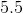Explanation:

To find the mode, you must find the most frequent number. Since all of the numbers are never repeated, there is no mode. Thus, our answer is "none".

### Example Question #113 : How To Find Mode

Find the mode of the following dataset: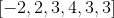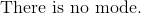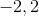Explanation:

The mode is defined as the number with the most occurrences in the dataset.

The only number that has the highest frequency is three.

The answer is:### Example Question #114 : How To Find Mode

Mr. Jesse recently graded his class's tests. He asked them to find the mode of the following scores. What is the correct answer?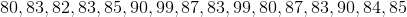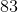Explanation:

The mode is the number that appears the most.Reorder the data set in ascending order.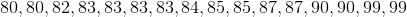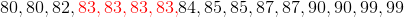was the most frequent score on the student's tests.

### Example Question #115 : How To Find Mode

Find the mode: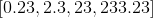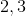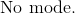Explanation:

The mode is the number or numbers in the dataset that have the highest frequency of occurrence.  Each number only appears once in the set, separated by a comma.  Do not mix up the definition of mode with the occurrence of digits in the dataset.

The answer is:### Example Question #116 : How To Find Mode

Find the mode of the following data set:

10, 9, 11, 10, 8, 4, 10, 7, 8Explanation:

The mode of a data set is the number that occurs most frequently.  Given the data set

10, 9, 11, 10, 8, 4, 10, 7, 8

we can see that the number 10 occurs most frequently.  Therefore, the mode of this data set is 10.

### Example Question #117 : How To Find Mode

Find the mode of the following data set:

13, 4, 6, 3, 9, 3, 11, 11, 3Explanation:

The mode of a data set is the number within the set that appears most frequently  Given the data set

13, 4, 6, 3, 9, 3, 11, 11, 3

The number 3 appears most frequently.  Therefore, the mode is 3.

### Example Question #118 : How To Find Mode

Find the mode: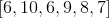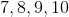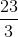Explanation:

The mode is the highest frequency number or numbers in a set.  The only number that appears more than once is.

The answer is:### Example Question #119 : How To Find Mode

Find the mode of the following set:

15, 9, 11, 14, 12, 11, 13, 11, 12Explanation:

To find the mode of a data set, you find the number that occurs most frequently.  In the data set

15, 9, 11, 14, 12, 11, 13, 11, 12

the number that appears most frequently is 11.

Therefore, the mode of the data set is 11.

### Example Question #120 : How To Find Mode

Find the mode of the following set: